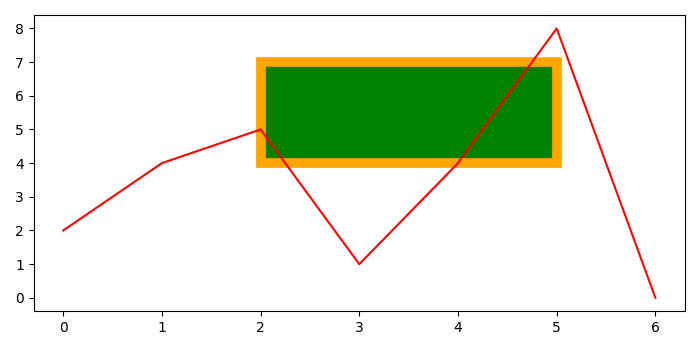# How to draw a rectangle over a specific region in a Matplotlib graph?

MatplotlibServer Side ProgrammingProgramming

To draw a rectangle over a specific region in a matplotlib graph, we can take the following steps −

• Using subplots() method, create a figure and a set of subplots, where nrows=1.

• Using rectangle, we can create a rectangle, defined via an anchor point and its width and height. Where, edgecolor=orange, linewidth=7, and facecolor=green.

• To plot a diagram over the axis, we can create a line using plot() method, where line color is red.

• To display the figure, use show() method.

## Example

from matplotlib import pyplot as plt, patches
plt.rcParams["figure.figsize"] = [7.00, 3.50]
plt.rcParams["figure.autolayout"] = True
figure, ax = plt.subplots(1)
rectangle = patches.Rectangle((2, 4), 3, 3, edgecolor='orange',
facecolor="green", linewidth=7)
ax.plot([2, 4, 5, 1, 4, 8, 0], c='red')
plt.show()